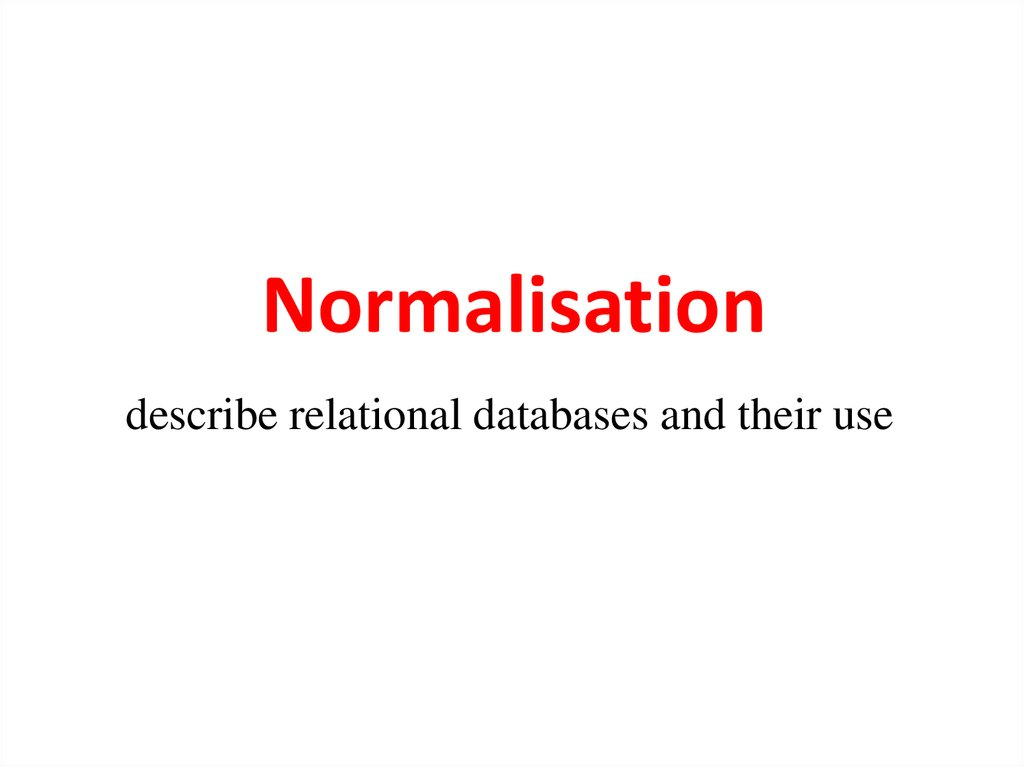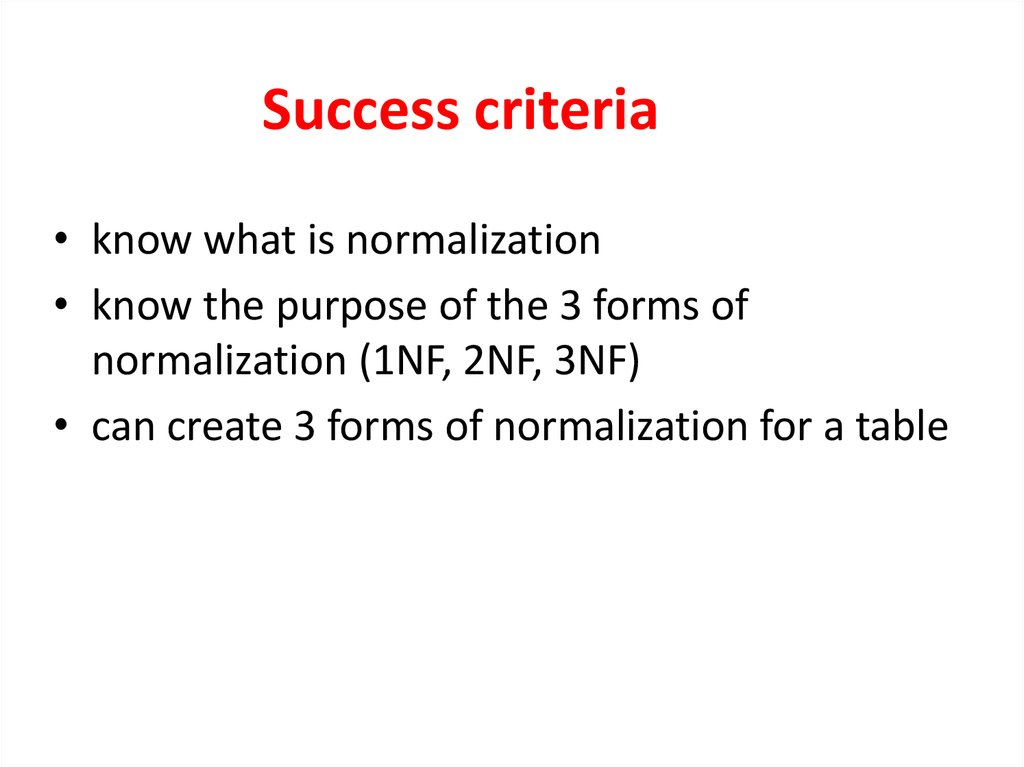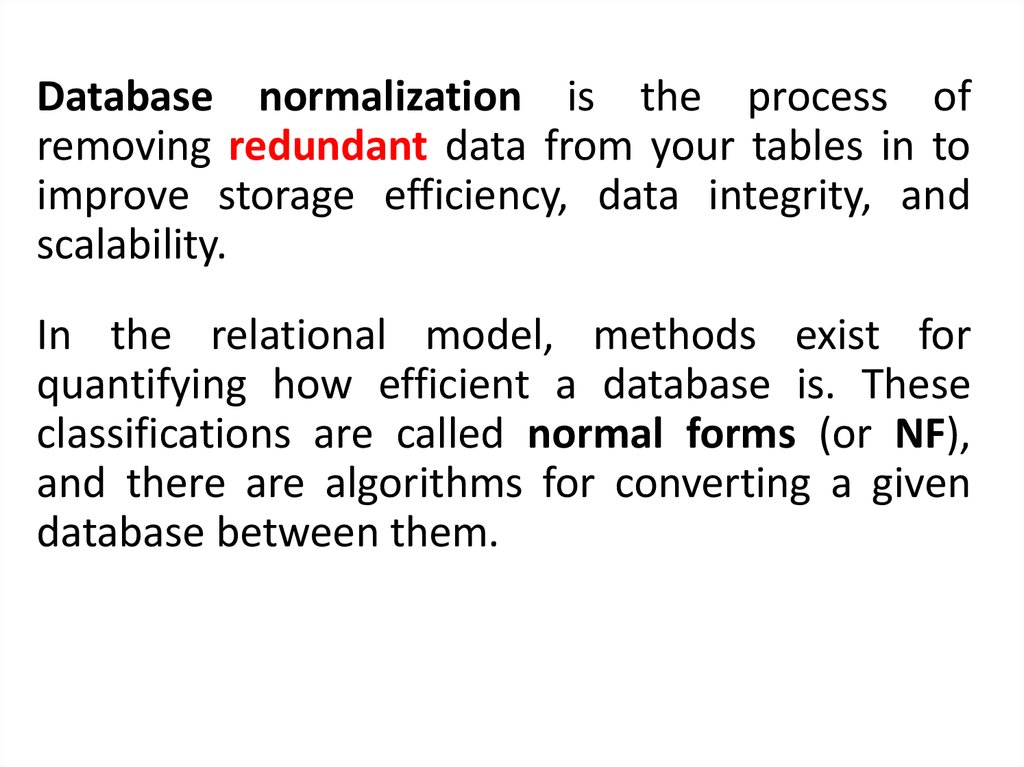# Normalisation. Describe relational databases and their use

## 1. Normalisation

describe relational databases and their use

## 2. Success criteria

• know what is normalization
• know the purpose of the 3 forms of
normalization (1NF, 2NF, 3NF)
• can create 3 forms of normalization for a table

## 3.

Database normalization is the process of
removing redundant data from your tables in to
improve storage efficiency, data integrity, and
scalability.
In the relational model, methods exist for
quantifying how efficient a database is. These
classifications are called normal forms (or NF),
and there are algorithms for converting a given
database between them.

## 4.

• 1NF - Atomic Data Test
If a table has a primary key it is said to be in First Normal form if the
table does not have repeating groups of attributes. All attributes
within the table need to be dependent only on the primary key.
• 2NF - Partial Dependence Test
For a table to be in Second Normal form it must first be in First
Normal (1NF) Form and then contain no data that is dependent on
only part of the Primary Key
• 3NF - Non-Key Dependence Test
For a table to be in Third Normal Form(3NF) it must be in Second
Normal form and contain No data that is not dependent on the
primary Key
e.g. (Remove columns that are not dependent upon the primary
key.)

Is it 1NF?

## 7. No. There are repeating groups (subject, subjectcost, grade)

How can you make it 1NF?

## 8. Create new rows so each cell contains only one value

But now look – is the studentID primary
key still valid?

## 9. No – the studentID no longer uniquely identifies each row

You now need to declare studentID and subject
together to uniquely identify each row.
So the new key is StudentID and Subject.

Is it 2NF?

## 11. Studentname and address are dependent on studentID (which is part of the key) This is good.

But they are not dependent on
Subject (the other part of the
key)

## 12. And 2NF requires…

All non-key fields are
dependent on the ENTIRE
key (studentID + subject)

## 13. So it’s not 2NF

How can we fix it?

## 14. Make new tables

• Make a new table for each primary key field
• Give each new table its own primary key
• Move columns from the original table to the
new table that matches their primary key…

## 15. Step 1

STUDENT TABLE (key = StudentID)

## 16. Step 2

STUDENT TABLE (key = StudentID)
SUBJECTS TABLE (key = Subject)

## 17. Step 3

STUDENT TABLE (key = StudentID)
SUBJECTS TABLE (key = Subject)
RESULTS TABLE (key = StudentID+Subject)

## 18. Step 3

STUDENT TABLE (key = StudentID)
SUBJECTS TABLE (key = Subject)
RESULTS TABLE (key = StudentID+Subject)

## 19. Step 4 - relationships

STUDENT TABLE (key = StudentID)
SUBJECTS TABLE (key = Subject)
RESULTS TABLE (key = StudentID+Subject)

## 20. Step 4 - cardinality

STUDENT TABLE (key = StudentID)
1
Each student can only appear
ONCE in the student table
RESULTS TABLE (key = StudentID+Subject)
SUBJECTS TABLE (key = Subject)

## 21. Step 4 - cardinality

STUDENT TABLE (key = StudentID)
1
SUBJECTS TABLE (key = Subject)
1
Each subject can only appear
ONCE in the subjects table
RESULTS TABLE (key = StudentID+Subject)

## 22. Step 4 - cardinality

STUDENT TABLE (key = StudentID)
1
SUBJECTS TABLE (key = Subject)
8
A subject can be listed MANY
times in the results table (for
different students)
RESULTS TABLE (key = StudentID+Subject)
1

## 23. Step 4 - cardinality

STUDENT TABLE (key = StudentID)
1
SUBJECTS TABLE (key = Subject)
8
8
A student can be listed MANY
times in the results table (for
different subjects)
RESULTS TABLE (key = StudentID+Subject)
1

## 24. A 2NF check

STUDENT TABLE (key = StudentID)
1
SUBJECTS TABLE (key = Subject)
8
8
1
RESULTS TABLE (key = StudentID+Subject)
SubjectCost is only
dependent on the
primary key,
Subject

## 25. A 2NF check

STUDENT TABLE (key = StudentID)
1
SUBJECTS TABLE (key = Subject)
8
8
1
on the primary key
(studentID + subject)
RESULTS TABLE (key = StudentID+Subject)

## 26. A 2NF check

STUDENT TABLE (key = StudentID)
8
dependent on the
primary key
(StudentID)
8
1
RESULTS TABLE (key = StudentID+Subject)
SUBJECTS TABLE (key = Subject)
1

## 27. But is it 3NF?

STUDENT TABLE (key = StudentID)
1
SUBJECTS TABLE (key = Subject)
1
8
8
So it is
2NF!
But is it 3NF?
RESULTS TABLE (key = StudentID+Subject)

## 28. A 3NF check

STUDENT TABLE (key = StudentID)
Oh oh…
8
What?
8
1
RESULTS TABLE (key = StudentID+Subject)
SUBJECTS TABLE (key = Subject)
1

## 29. A 3NF check

STUDENT TABLE (key = StudentID)
8
HouseName is
dependent on both
StudentID +
HouseColour
8
1
RESULTS TABLE (key = StudentID+Subject)
SUBJECTS TABLE (key = Subject)
1

## 30. A 3NF check

STUDENT TABLE (key = StudentID)
8
Or HouseColour is
dependent on both
StudentID +
HouseName
8
1
RESULTS TABLE (key = StudentID+Subject)
SUBJECTS TABLE (key = Subject)
1

## 31. A 3NF check

STUDENT TABLE (key = StudentID)
8
But either way,
non-key fields are
dependent on MORE
THAN THE PRIMARY
KEY (studentID)
8
1
RESULTS TABLE (key = StudentID+Subject)
SUBJECTS TABLE (key = Subject)
1

## 32. A 3NF check

STUDENT TABLE (key = StudentID)
8
And 3NF says that
non-key fields must
depend on nothing
but the key
8
1
RESULTS TABLE (key = StudentID+Subject)
SUBJECTS TABLE (key = Subject)
1

## 33. A 3NF check

STUDENT TABLE (key = StudentID)
1
8
8
WHAT DO
WE DO?
RESULTS TABLE (key = StudentID+Subject)
SUBJECTS TABLE (key = Subject)
1

## 34. Again, carve off the offending fields

1
8
8
SUBJECTS TABLE (key = Subject)
RESULTS TABLE (key = StudentID+Subject)
1

## 35. A 3NF fix

1
8
8
SUBJECTS TABLE (key = Subject)
RESULTS TABLE (key = StudentID+Subject)
1

## 36. A 3NF fix

8
A 3NF fix
1
1
8
8
SUBJECTS TABLE (key = Subject)
RESULTS TABLE (key = StudentID+Subject)
1

## 37. A 3NF win!

8
A 3NF win!
1
8
8
1
1
SUBJECTS TABLE (key = Subject)
RESULTS TABLE (key =
StudentID+Subject)
Or…

## 38. The Reveal

Before…
After…
8
1
1
8
8
1
SUBJECTS TABLE (key = Subject)
RESULTS TABLE (key = StudentID+Subject)